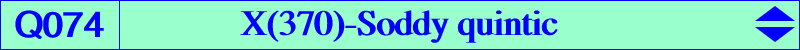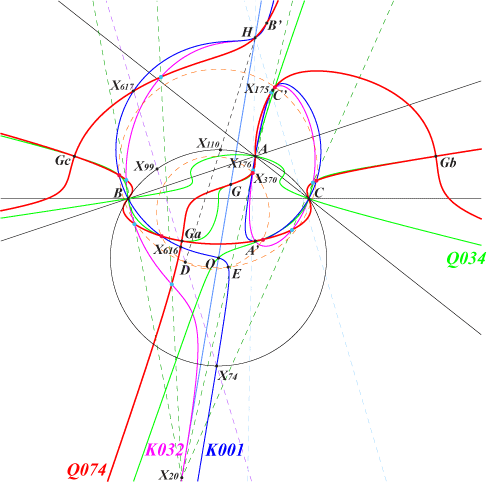too complicated to be written here. Click on the link to download a text file.X(2), X(4), X(175), X(176), X(370), X(616), X(617) 6 other Soddy centers, the extraversions of X(175), X(176) 5 other equilateral cevian points, see table 10 anticomplements of the 6 equilateral cevian points Ga, Gb, Gc vertices of the antimedial triangle Ga', Gb', Gc' their reflections about A, B, C A', B', C' reflections of A, B, C in the sidelines of ABC S1, S2, S3, S4 on the Kiepert hyperbola details and figures belowQ074 is the anticomplement of Q034. It is a quintic passing through the equilateral cevian point, their anticomplements and a large number of other points as seen below. Q074 passes through the vertices of ABC which are three inflexion points. The inflexional tangents are the altitudes of ABC. Q074 passes through the vertices Ga, Gb, Gc of the antimedial triangle which are three nodes. The nodal tangents at Ga are parallel to the bisectors at A of ABC. The tangent at G is the line GK.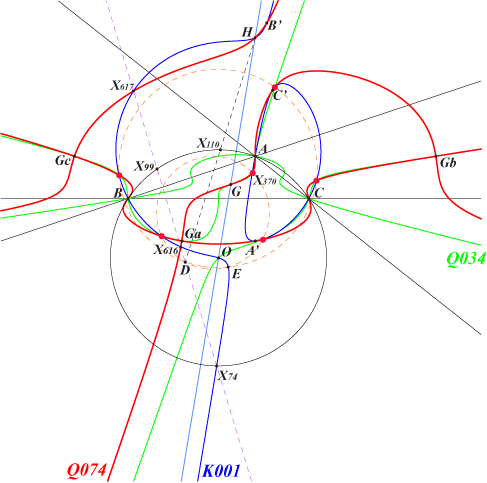Equilateral cevian points These are X(370) and its five mates described in Table 10. The six (red) points lie on the Neuberg cubic K001 and several other curves such as Q034. The 15 common points of Q074 and K001 are A, B, C, A', B', C', H, X(616), X(617) and these six points.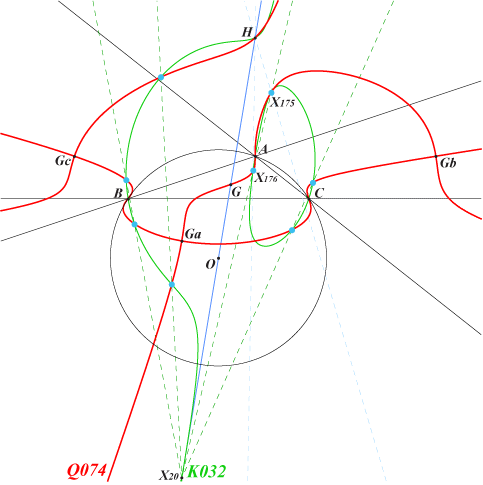Soddy centers There are eight Soddy centers : X(175), X(176) and their six extraversions. They lie on the lines passing through the de Longchamps point X(20) and the in/excenters of ABC. These (light blue) points also lie on the Soddy cubic K032.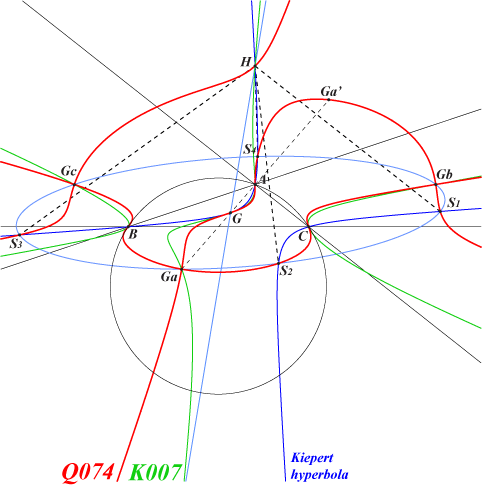Points S1, S2, S3, S4 These are the common points of the Kiepert hyperbola and the antimedial Steiner ellipse. Indeed, the barycentric equation of Q074 can be written under the form : [∑(x^2+3yz)] [∑a^2(x+y)(x+z)(y-z)] - 2(x+y)(y+z)(z+x) [∑(b^2-c^2)yz] = 0 These four points are the feet of the normals dropped from H to this ellipse hence the Kiepert hyperbola is the Apollonius hyperbola of H with respect to this ellipse (theorem of Joachimstahl).Anticomplements of the CPCC points The CPCC points are the four last common points of the Darboux cubic K004 and the Lucas cubic K007. See Table 11. Their anticomplements lie on Q074, the anticomplements of the cubics K004, K007, K199 and many other cubics. These also lie on the rectangular hyperbola (H) passing through O, X(69), X(185), X(193), X(2574), X(2575), X(3146).